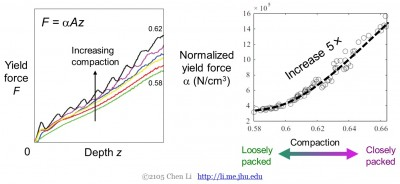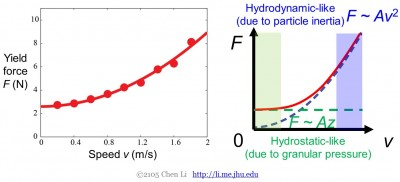# Globalized vs. Localized Forcing

A large body of literature has studied the liquid-like dense granular flows and solid-fluid (jamming and unjamming) transitions. Much focus is on the physics of globalized forcing dominant in applications involving material handling (e.g., pharmaceutical, agriculture, energy production), where a large bulk of granular media are forced and undergo movement together.

During movement of animals and robots on granular media like sand, however, particles move only locally around small intruders (e.g., feet and legs) while the bulk of granular material stays like a solid. The physics of such localized forcing in granular media is less explored.

Using our fluidized beds to control granular media and robotic arm to control intruders (Fig. 1), we systematically studied granular intrusion forces as a function of both intruder parameters (e.g., speed, orientation, movement direction, depth) and particle parameters (compaction, size, density, polydispersity).

# Force vs. Depth, Compaction, and Speed

We first focus on the physics of intrusion forces at low intruder speeds (< 0.5 m/s). For an intruder moving in granular media of a given compaction, we found that force is roughly proportional to intruder area (projected into the horizontal plane that is perpendicular to velocity), and is proportional to intruder depth (Fig. 2, left). This scaling with intruder area is because force is exerted by the material in front of the moving intruder pushes back against it.

We also discovered that normalized force (force per unit area and depth) increases with particle compaction by up to 5 times over naturally occurring compaction ranges (Fig. 2, right).Figure 2. Granular intrusion force is proportional to intruder area, depth, and increases with particle compaction. For the data shown, intruder is a horizontal plate penetrating downward.

We then studied how intrusion force depends on intruder speed. We found that at low speeds (< 0.5 m/s), force is insensitive to intruder speed, but at high speeds (> 1 m/s), force increases roughly quadratically with speed (Fig. 3, left).Figure 3. Granular intrusion force is insensitive to speed at low intruder speeds, but increases quadratically at higher speeds.

# Frictional vs. Inertial Fluid Regimes

Based on these observations, we can understand granular intrusion force using a simple physics picture (Fig. 3, right).

For an intruder moving within incompressible but flowable media, material that is being accelerated away from the intruder can exert an inertial force on it. From simple calculations we know that inertial force is quadratic to intruder speed.

At low enough speeds (<0.5 m/s), inertial force is negligible. In this case, force on an intruder in granular media is dominated by friction. In granular media, there exists a hydrostatic-like pressure that is proportional to depth (i.e. normal force is proportional to depth). So friction is also proportional to depth. Therefore, granular media behave like a “frictional fluid” in this regime. This is analogous to fluids at low Reynolds number, except that it is frictional force rather than viscous force that dominates.

At high enough speeds (> 1 m/s), however, inertial force becomes dominant. This is similar to fluids at high Reynolds number. Therefore, granular media behave like a “inertial fluid” in this regime.

The small animals and robots that we study turn out to move their body and legs slowly enough (<0.5 m/s) in most cases that they primarily experience frictional forces. This makes movement in granular media at low speeds analogous to swimming in fluids at low Reynolds number, as in both cases inertia of the media is negligible.

Related publications:

1. Li C, Umbanhowar PB, Komsuoglu H, Koditschek DE, Goldman DI (2009). Sensitive dependence of the motion of a legged robot on granular media, Proceedings of the National Academy of Sciences, 106, 3029-3034 (Featured in IEEE Spectrum and Physics Today; Best Student Paper in DCB at SICB 2009) PDF
2. Li C, Umbanhowar PB, Komsuoglu H, Goldman DI (2010). The effect of limb kinematics on the speed of a legged robot on granular media, Experimental Mechanics, 50, 1383-1393 PDF
3. Li C, Hsieh ST, Goldman DI (2012). Multi-functional foot use during running in the zebra-tailed lizard (Callisaurus draconoides), Journal of Experimental Biology, 215, 3293-3308 (Cover Article) PDF
4. Maladen RD, Ding Y, Li C, Goldman DI (2009). Undulatory swimming in sand: subsurface locomotion of the sandfish lizard, Science, 325, 314-318 (Featured in Nature News & Views) PDF